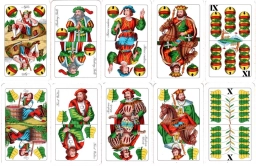# Divided 81763

Petr divided the 32 playing cards into two piles. He found that one of them had three times as many cards as the other. How many cards were in each pile?

a =  24
b =  8

### Step-by-step explanation:Did you find an error or inaccuracy? Feel free to write us. Thank you!

Tips for related online calculators
Do you have a linear equation or system of equations and looking for its solution? Or do you have a quadratic equation?